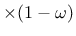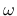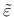### Isotropic damage model for interfaces using tabulated data for damage

The model provides an interface law which can be used to describe a damageable interface between two materials (e.g. between steel reinforcement and concrete). The law is formulated in terms of the traction vector and the displacement jump vector. The basic response is elastic, with stiffness kn in the normal direction and ks in the tangential direction. Similar to other isotropic damage models, this model assumes that the stiffness degradation is isotropic, i.e., both stiffness moduli decrease proportionally and independently of the loading direction. The damaged stiffnesses are knand kswhereis a scalar damage variable.

The equivalent “strain”,, is a scalar measure derived from the displacement jump vector. The choice of the specific expression for the equivalent strain affects the shape of the elastic domain in the strain space and plays a similar role to the choice of a yield condition in plasticity. Currently, in the present implementation,is equal to the positive part of the normal displacement jump (opening of the interface).

The damage evolution law is postulated in a separate file that should have the following format. Each line should contain one strain, damage pair separated by a whitespace character. The exception to this is the first line which should contain a single integer stating how many strain, damage pairs that the file will contain. The strains given in the file is defined as the equivalent strain minus the limit of elastic deformation. To find the damage for arbitrary strains linear interpolation between the tabulated values is used. If a strain larger than one in the given table is achieved the respective damage for the largest tabulated strain will be used. Both the strains and damages must be given in a strictly increasing order.

The model parameters are summarized in Tab. 29.

Table 29: Isotropic damage model for interface elements using tabulated data for damage - summary.
 Description Isotropic damage model for concrete in tension Record Format isointrfdm02 kn(rn) # ks(rn) # ft(rn) # tablename(rn) # [ maxomega(rn) #] talpha(rn) # d(rn) # Parameters - d material density - tAlpha thermal dilatation coefficient - kn elastic stifness in normal direction - ks elastic stifness in tangential direction - ft tensile strength - tablename file name of the table with the strain damage pairs - maxomega maximum damage, used for convergence improvement (its value is between 0 and 0.999999 (default), and it affects only the secant stiffness but not the stress) Supported modes 2dInterface, 3dInterface Features

Borek Patzak
2019-03-19